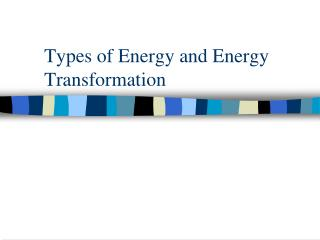DownloadDownload PresentationTypes of Energy and Energy Transformation

# Types of Energy and Energy Transformation

Télécharger la présentation## Types of Energy and Energy Transformation

- - - - - - - - - - - - - - - - - - - - - - - - - - - E N D - - - - - - - - - - - - - - - - - - - - - - - - - - -
##### Presentation Transcript

1. Types of Energy and Energy Transformation

2. Recap • Energy is the ability of a system or object to change or do work. • Work is done when a force is applied to an object and the object moves as a result of the force.

3. Types of Energy • While there are many types of energy used to power the world, we are going to look at the 6 main types of energy. • Types of Energy • Thermal • Mechanical • Electrical • Light • Sound • Chemical

4. Thermal (Heat) Energy • Energy that is associated with the random motion and arrangement of the particles of a material. • The more the particles are moving, the higher the thermal energy. • Examples: radiation, stoves, matches

5. Mechanical Energy • Is the energy due to the position of something or the movement • It can be in the form of potential energy, kinetic energy or a sum of the two put together. • Examples: walking, running, moving vehicles, moving turbines etc.

6. Electrical Energy • Is associated with current and voltage. • Examples: Plugging into an outlet, power plants, anything that uses electricity involves electrical energy.

7. Light Energy • Is energy associated with the electromagnetic waves. • Largest source is the sun • Examples: Plants use it to make food and scientists use it to create lasers

8. Sound Energy • Energy that is associated with longitudinal mechanical waves. • Travels from the source in the form of waves. • Examples: Radio, sirens, alarm clock, ipod, etc.

9. Chemical Energy • Energy associated with atoms, ions, and molecules and the bonds that they form. • Chemical energy will change into another type of energy when a chemical reaction occurs. • Examples: Breathing, photosynthesis, digestion etc

10. Energy Transformation when a battery is connected to a light bulb • Chemical energy changes to electrical energy. • The electrical energy flows through the light bulb and turns electrical energy to light and thermal energy. • The total of the energy from the chemical reaction in the battery is equal to the total energy that it transforms into.

11. Energy Transformation when throwing a football • A football player converts chemical energy from the food he/she has eaten to mechanical energy when he/she moves his/her arm to throw the ball. • The work done on the ball converts the energy of the arm movement to kinetic mechanical energy of the moving ball. • As the ball moves through the air, it has both kinetic and potential mechanical energy.

12. When a second player catches the ball, the ball does work on the player’s hand giving them some mechanical energy. • The ball also moves the molecules in their hand moving them faster and thus heating their hand. • The player that catches the ball absorbs the energy of the ball, and this energy turns to heat. • The total heat produced is equal to the energy used to throw the ball.

13. Max SOUND energy